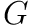### Path 3-coloring plane graphs

Posted on June 15, 2018

A path coloring of a graph partitions its vertex set into color classes such that each class induces a disjoint union of paths. In my computer science masters project I implemented several algorithms to compute path colorings of graphs embedded in the plane.

### Abstract

We present two algorithms to path color plane graphs with 3 colors based on a proof by Poh in 1990. First we consider a naive algorithm that directly follows Poh’s procedure, then we modify the algorithm to run in linear time.

Independent results of Hartman and Skrekovski describe a procedure that takes a plane graphand a list of 3 colors for each vertex, and computes a path coloring ofsuch that each vertex receives a color from its list. We implement a linear time algorithm based on Hartman and Skrekovski’s proofs.

A C++ implementation is provided for all three algorithms, utilizing the Boost Graph Library. Instructions are given on how to use the implementation to construct colorings for plane graphs represented by Boost data types.